# Multiplication Problems

In this worksheet, students must solve multiplication problems.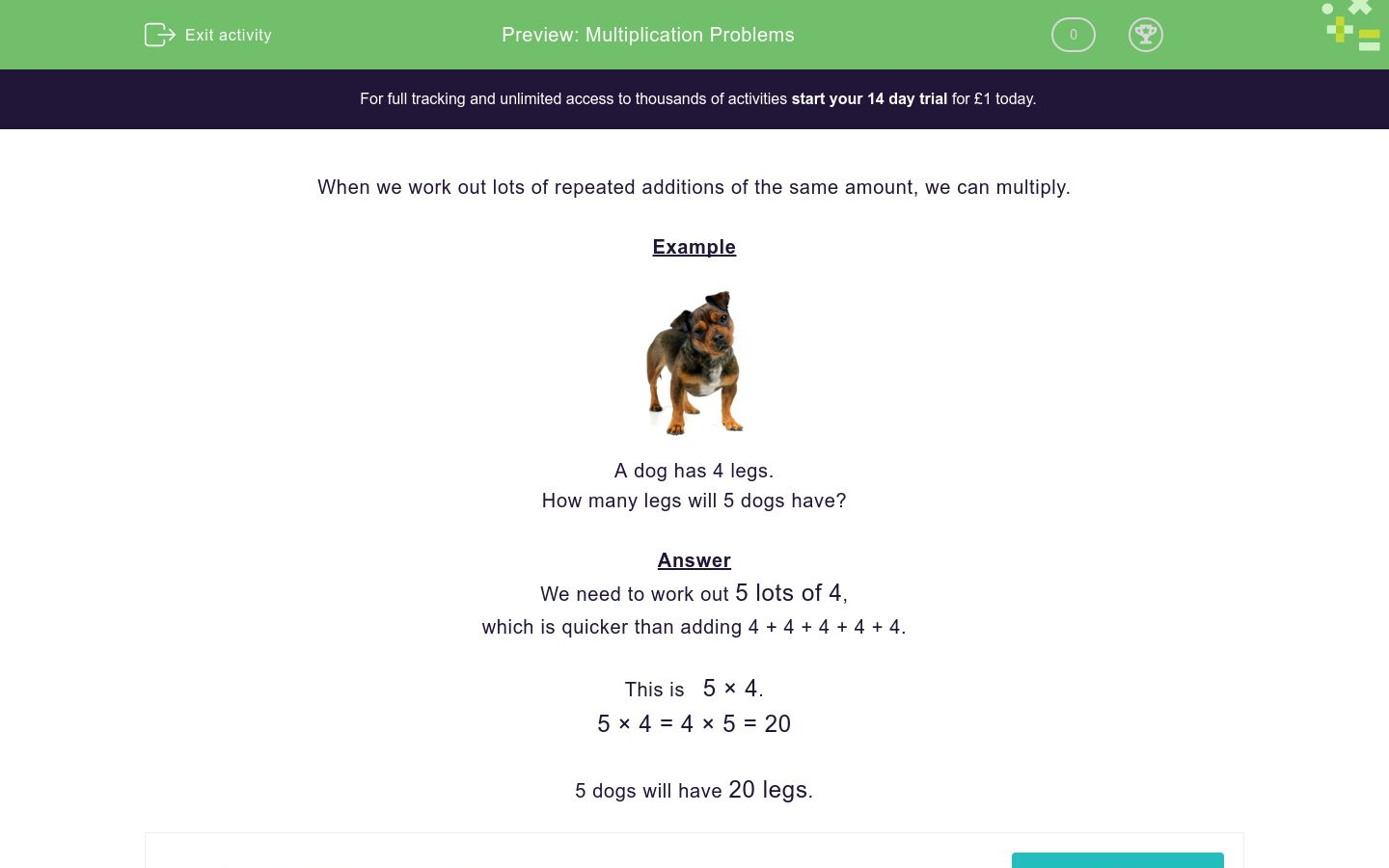Key stage:  KS 1

Curriculum topic:   Number: Multiplication and Division

Curriculum subtopic:   Solve Problems Using Multiplication/Division

Difficulty level:### QUESTION 1 of 10

When we work out lots of repeated additions of the same amount, we can multiply.

Example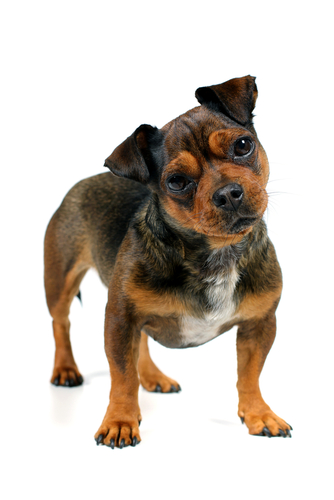A dog has 4 legs.

How many legs will 5 dogs have?

We need to work out 5 lots of 4,

which is quicker than adding 4 + 4 + 4 + 4 + 4.

This is   5 × 4.

5 × 4 = 4 × 5 = 20

5 dogs will have 20 legs.A bird has 2 wings.

How many wings will 6 birds have?A star has 5 points.

How many points will 6 stars have?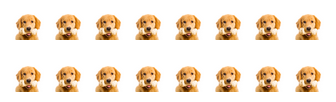Here are 2 rows of dogs.

There are 8 dogs in each row.

Use multiplication to work out the total number of dogs.A cake has 10 candles.

How many candles will 7 of the same cakes have?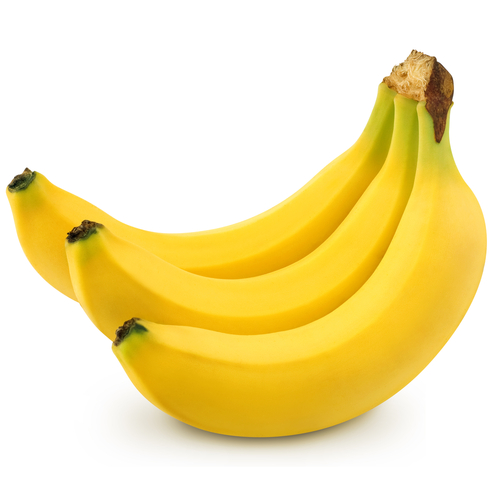A plate has 3 bananas.

How many bananas will there be on 5 similar plates?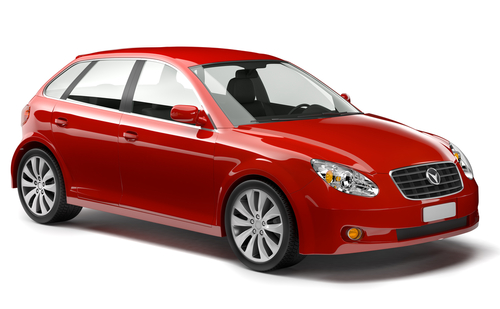A car has 4 wheels.

How many wheels will there be on 5 cars?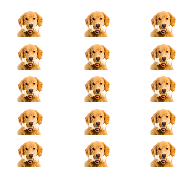Here are 5 rows of dogs.

There are 3 dogs in each row.

Use multiplication to work out the total number of dogs.Apples cost 10p each.

How much will 9 apples cost in pence?

(Just write the number.)Here are 5 rows of dogs.

There are 10 dogs in each row.

Use multiplication to work out the total number of dogs.This flower has 5 petals.

How many petals will there be on 9 similar flowers?

• Question 1A bird has 2 wings.

How many wings will 6 birds have?

12
EDDIE SAYS
6 × 2 = 12 wings
• Question 2A star has 5 points.

How many points will 6 stars have?

30
EDDIE SAYS
6 × 5 = 30 points
• Question 3Here are 2 rows of dogs.

There are 8 dogs in each row.

Use multiplication to work out the total number of dogs.

16
EDDIE SAYS
2 × 8 = 16 dogs
• Question 4A cake has 10 candles.

How many candles will 7 of the same cakes have?

70
EDDIE SAYS
7 × 10 = 70 candles
• Question 5A plate has 3 bananas.

How many bananas will there be on 5 similar plates?

15
EDDIE SAYS
5 × 3 = 15 bananas
• Question 6A car has 4 wheels.

How many wheels will there be on 5 cars?

20
EDDIE SAYS
5 × 4 = 20 wheels
• Question 7Here are 5 rows of dogs.

There are 3 dogs in each row.

Use multiplication to work out the total number of dogs.

15
EDDIE SAYS
5 × 3 = 15 dogs
• Question 8Apples cost 10p each.

How much will 9 apples cost in pence?

(Just write the number.)

90
EDDIE SAYS
9 × 10 = 90p
• Question 9Here are 5 rows of dogs.

There are 10 dogs in each row.

Use multiplication to work out the total number of dogs.

50
EDDIE SAYS
5 × 10 = 50 dogs
• Question 10This flower has 5 petals.

How many petals will there be on 9 similar flowers?

45
EDDIE SAYS
9 × 5 = 45 petals
---- OR ----

Sign up for a £1 trial so you can track and measure your child's progress on this activity.

### What is EdPlace?

We're your National Curriculum aligned online education content provider helping each child succeed in English, maths and science from year 1 to GCSE. With an EdPlace account you’ll be able to track and measure progress, helping each child achieve their best. We build confidence and attainment by personalising each child’s learning at a level that suits them.

Get started